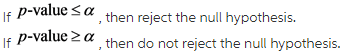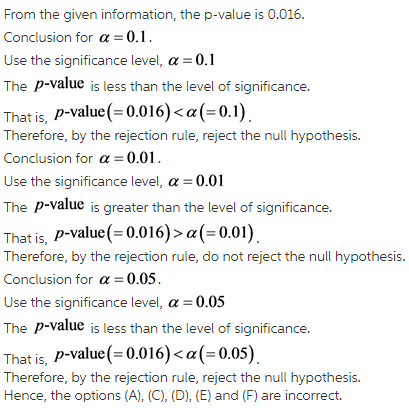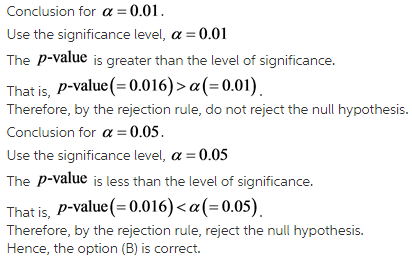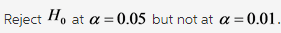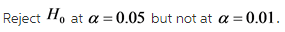# Suppose you conduct a test and your p-value is equal to 0.016. What can you conclude?

Suppose you conduct a test and your p-value is equal to 0.016. What can you conclude?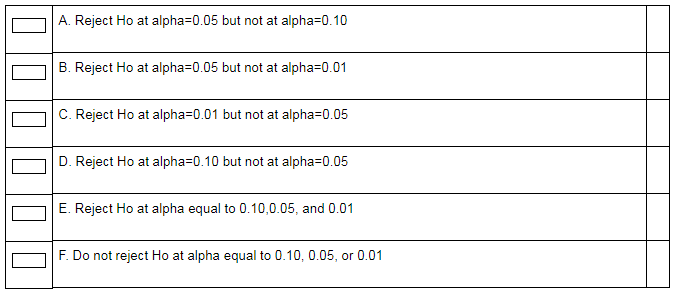Concepts and reason
Null hypothesis: The null hypothesis states that there is no difference in the test, which is denoted by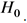Moreover, the sign of null hypothesis is equal (=), greater than or equalAlternative hypothesis: The hypothesis that differs from theis called alternative hypothesis. This signifies that there is a significant difference in the test. The sign of alternative hypothesis is less than (<), greater than (>), or not equal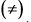P-value: The probability of getting the value of the statistic that is as extreme as the observed statistic when the null hypothesis is true is called as P-value.

Fundamentals
Rejection rule based on p-value: